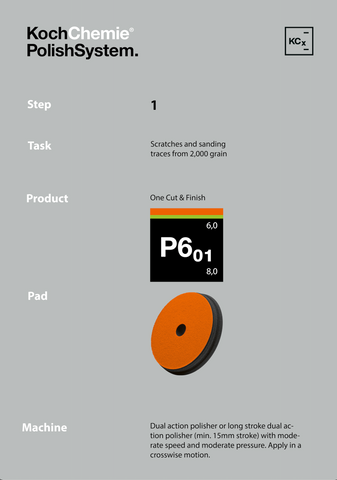# Our SystemBasic Size Information:

250 mL (milliliter) = 8.4 fl oz = About 1/2 Pint

500 mL (milliliter) = 16.9 fl oz = About 1 Pint

1 L (liter) = 33.8 fl oz = About 1 Quart

5 L (liter) = 169.7 fl oz = About 1.3 Gallon